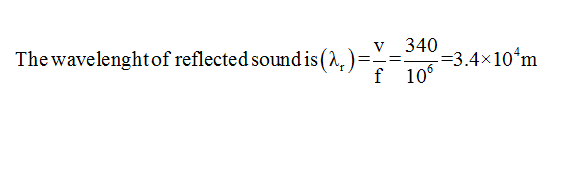# A bat emits ultrasonic sound of frequency 1000 kHz in air. If the sound meets a water surface, what is the wavelength of (a) the reflected sound, (b) the transmitted sound?Speed of sound in air is 340 m s-1 and in water 1486 m s-1

Question
2 views

A bat emits ultrasonic sound of frequency 1000 kHz in air. If the sound meets a water surface, what is the wavelength of (a) the reflected sound, (b) the transmitted sound?Speed of sound in air is 340 m s-1 and in water 1486 m s-1

check_circle

Step 1

Given:

a)

Frequecy of the ulyrasonic sound (f)=1000Hz=106Hz

Speed of sound in the air (Va)340m/s

Step 2

Calculation:Step 3

b)

Given :

f=1000Hz=106Hz

Speed of sound in wate...

### Want to see the full answer?

See Solution

#### Want to see this answer and more?

Solutions are written by subject experts who are available 24/7. Questions are typically answered within 1 hour.*

See Solution
*Response times may vary by subject and question.
Tagged in